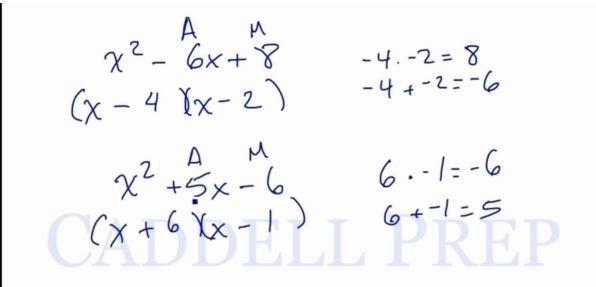In this video, we are going to look at how to factor quadratics. After you finish this lesson, view all of our Algebra 1 lessons and practice problems.

Quadratics are written in the form$ax^2+bx+c$. When factoring these, it will be split into two binomials, both of which will begin with x (when a is 1), and have the second terms add up to equal b, and multiply to equal c
For example:
To factor$x^2+6x+8$ we have to first write two sets of parentheses beginning with an x. Then, we have to find two numbers that will add up to equal 6, and multiply to equal 8. These two numbers are 2 and 4, so when we factor this quadratic, we get$(x+2)(x+4)$

To factor$x^2-6x+8$ we solve in the same way, only now b is negative. If two numbers add up to be negative but multiply to be positive then we know that both numbers are going to be negative. These two numbers are -4 and -2, so when we factor this quadratic, we get$(x-4)(x-2)$

To factor$x^2+5x-6$ we solve in the same way, only now c is negative. If two numbers add up to be positive but multiply to be negative then we know the signs have to be different. These two numbers are 6 and -1, so when we factor this quadratic, we get$(x+6)(x-1)$*Note that when the signs are different, the number with the larger absolute value will have the same sign as the middle term of the quadratic.

To factor$x^2-4x-21$ we solve in the same way, only now both b and c are negative. If two numbers add up to be negative, and multiply to be negative then we know the signs have to be different. These two numbers are -7 and 3, so when we factor this quadratic, we get$(x-7)(x+3)$

### Example 1

Factor$x^2 + 13x + 40$
Let’s write two parenthesis and an$x$ in each parenthesis.

Now, what numbers will add up to equal$13$ and when multiplied equals$40$?$8$ and$5$
Where:$8+5=13$
and$8\times5=40$$(x + 8) (x + 5)$
or$(x+5)(x+8)$

### Example 2

Factor$x^2 - 2x - 24$
Let’s write two parenthesis and an$x$ in each parenthesis.
Since the second and third terms are both negatives, our sign should be positive and negative.
So, what numbers should we add up to get$-2$ and when multiplied will get$-24$?$-6$ and$4$
Where:$-6+4=-2$
and$-6 \times 4 = -24$
and
Here we need to end up in a negative, so the bigger number should also be negative.$(x+4)(x-6)$

## Video-Lesson Transcript

Let’s go over how to factor quadratics.

A quadratic is$ax^2 + bx + c$.

And we’ll focus on quadratics where$a = 1$.

So, we really have$x^2 + bx + c$

When we factor this, we’ll have two$x$ to result in$x^2$.

And we also need a number to get inside the parenthesis so that we’ll have$bx + c$.

These numbers should add up and equal$bx$.

And when multiplied, will give us$c$.

Let’s have an example:$x^2 + 6x + 8$

Let’s write two parenthesis and an$x$ in each.

Now, what numbers will add up to equal$6$ and when multiplied equals$8$?$2$ and$4$$(x + 2) (x + 4)$

It doesn’t matter if you write this as$(x + 4) (x + 2)$

We’ll still have the same answer.

Let’s try this one.$x^2 - 6x + 8$

Looks similar but the only difference is that we now have a negative.

We will follow the same rules, though.

Let’s write two parenthesis and an$x$ in each parenthesis.

Let’s write letter “A” at the top of$- 6x$, to represent addition and “M” above$+ 8$ representing multiplication.

So now, what numbers will add up and give us$- 6$ and at the same time will give us$+ 8$ when multiplied.

To come up with a positive sign, we should have two positives or two negatives to result in a positive.

But since$- 6$ has a negative sign, we cannot have both positive numbers. Two positives will not result to a negative number.

So we’ll have two negative numbers.

What numbers will we add up to get$- 6$ and when multiplied will give us$+ 8$?$- 4$ and$- 2$

Look!$-4 \times -2 = 8$
and$-4 + -2 = -6$$(x - 4) (x - 2)$

Let’s try a different one.$x^2 + 5x - 6$

This one is a little bit different since the last term is negative and the second term is positive.

Since we have the third term in a negative sign, we have to have different signs in our answer.

Now, what numbers will we add up to get$+ 5$ and when multiplied will give us$- 6$?

That’s$6$ and$- 1$.

Let’s see.$6 \times -1 = -6$
and$6 + -1 = 5$

You have to keep in mind that since we should end up with a positive number, the bigger number should also be positive.$(x + 6) (x - 1)$

If you’re wondering how this works out, we just have to distribute it and we should have the same thing.

Let’s multiply this back out.$(x + 6) (x - 1)$$x^2 - x + 6x - 6$

Combine like terms$x^2 + 5x - 6$

Because we got the same thing, this means that we got the correct answer in factoring.

Let’s factor this one.$x^2 - 4x - 21$

Let’s write two parenthesis and an$x$ in each parenthesis.

Since the second and third terms are both negatives, our sign should be positive and negative.

So, what numbers should we add up to get$- 4$ and when multiplied will get$- 21$?$7$ and$3$

Here we need to end up in a negative, so the bigger number should also be negative.$(x + 3) (x - 7)$$3 \times -7 = -21$$3 + -7 = -4$